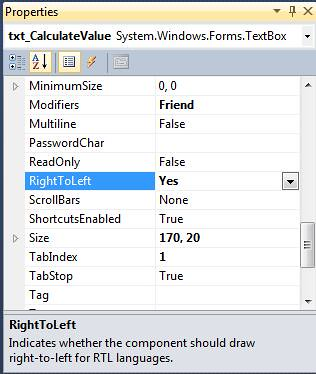# VB.net Create Button Dynamically—VB.Net 用程式碼建立按鈕

TextBox 的名稱是 “txt_CalculateValue

RightToLeft 屬性 設定成 “TrueButton 的名稱是”btn_Del

[vb]
Public Class ShareChiWaiCalculator

‘建立了一個按鈕給大家刪去一些在txt_CalculateValue的文字
Private Sub btn_Del_Click(ByVal sender As System.Object, ByVal e As System.EventArgs) Handles btn_Calculate.Click
If txt_CalculateValue.Text.Length > 0 Then
txt_CalculateValue.Text = txt_CalculateValue.Text.Substring(0, txt_CalculateValue.Text.Length – 1)
End If

End Sub

Public Sub CreateSimpleCalculatorButton()
‘建立 坐標位置的變數, 方便一會兒安排 自動生產的button 的位置
Dim y As Integer = 10
Dim x As Integer = 0

‘ 用for loop 來建立 這些button 做 10個button
‘因為多數的計算機的數字只由下至上排列的, 所以我便用了
‘For Loop 中的Step – 1 來 建立我的動態按鈕…[比較容易一點]
For ButtonIndex As Integer = 9 To 0 Step -1

‘ 建造新的 button
Dim CalculatorButton As New Button
‘設定這新的button 的闊度
CalculatorButton.Width = 35
‘設定這新的button 的高度
CalculatorButton.Height = 35

‘我們暫定每行有3個BUTTON, 當每行有多個4個BUTTON 時重設行距, 令到下一行的和這一行的距離有10 個PIXEL
‘ 和重設x 坐標` = 0, 令到位置由頭開始

If ButtonIndex Mod 3 = 0 Then
‘設定 TOP 的 坐標位置令他可以和上一排的有10個PIXEL 的距離
y += CalculatorButton.Height + 10
x = 0
End If

‘設定文字 和 位置
CalculatorButton.Text = ButtonIndex

‘設定 TOP 的 坐標位置
CalculatorButton.Top = y
‘下面的CODE 可以令到這個BUTTON 和另一個BUTTON 有5個PIXEL 的距離
CalculatorButton.Left = 26 + (x * (CalculatorButton.Width + 5))
x += 1
‘設定 BUTTON CLICK EVENT

‘把button 加到 form 中
Next
End Sub

‘每當使用者按下這些按鈕時, 他便會打 按鈕上的文字寫到 上面的TextBox 上 [txt_CalculateValue]
Private Sub CalculatorButton_Click(ByVal sender As Object, ByVal e As EventArgs)
Dim btn As Button = sender
txt_CalculateValue.Text &= btn.Text

End Sub

Private Sub ShareChiWaiCalculator_Load(ByVal sender As System.Object, ByVal e As System.EventArgs) Handles MyBase.Load
CreateSimpleCalculatorButton()
End Sub
End Class
[/vb]

Hope you find it useful, 歡迎大家給我一些意見, 令我可以發多一些有用的文章/教學

This site uses Akismet to reduce spam. Learn how your comment data is processed.